## Over 20 formulas packed into one app to help save you a lot of time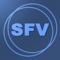# SuperFVCalc: FV, PV, Annuities

by RIEU Limited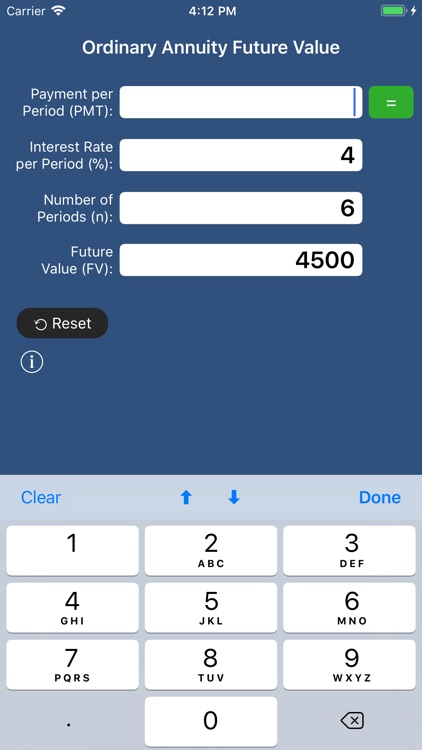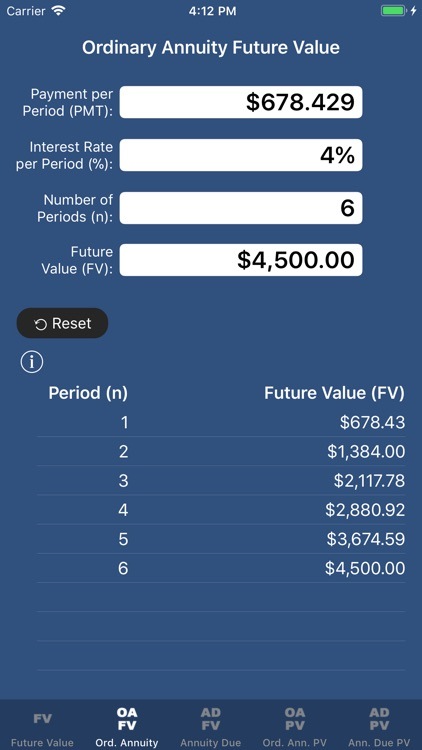Over 20 formulas packed into one app to help save you a lot of time! Calculate any variable for future values or annuities.### App Details

Version
1.1.3
Rating
(42)
Size
14Mb
Genre
Last updated
April 18, 2019
Release date
February 26, 2018

### App Screenshots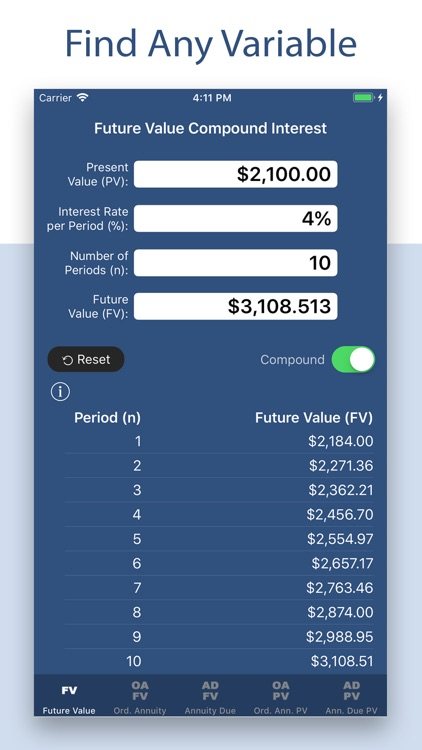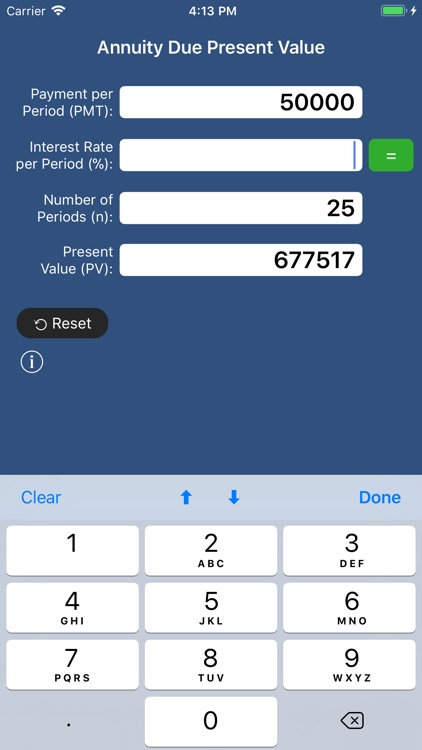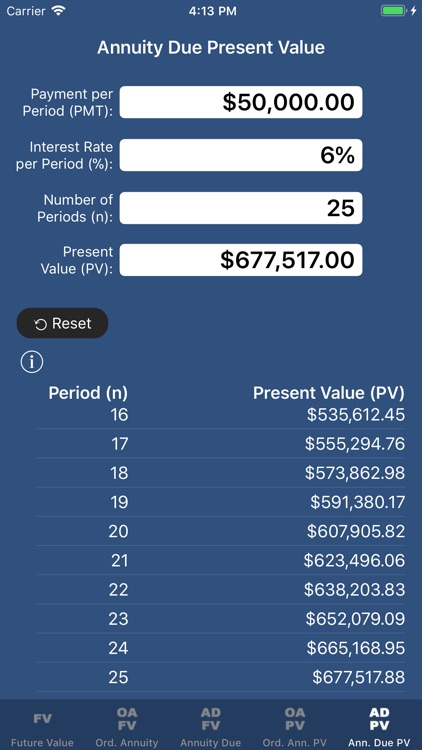### App Store Description

Over 20 formulas packed into one app to help save you a lot of time! Calculate any variable for future values or annuities.

Future Value Compound/Simple Interest calculator:

• Calculate FV from PV, Interest Rate and Number of Periods.
• Calculate PV from FV, Interest Rate and Number of Periods.
• Calculate Interest Rate from PV, FV and Number of Periods.
• Calculate Number of Periods from PV, FV and Interest Rate.

Ordinary Annuity Future Value calculator:

• Calculate FV from Payment per Period, Rate and Number of Periods.
• Calculate Payment per Period from FV, Rate and Number of Periods.
• Calculate Rate from FV, Payment per Period and Number of Periods.
• Calculate Number of Periods from FV, Payment per Period and Rate.

Annuity Due Future Value calculator:

• Calculate FV from Payment per Period, Rate and Number of Periods.
• Calculate Payment per Period from FV, Rate and Number of Periods.
• Calculate Rate from FV, Payment per Period and Number of Periods.
• Calculate Number of Periods from FV, Payment per Period and Rate.

Ordinary Annuity Present Value calculator:

• Calculate PV from Payment per Period, Rate and Number of Periods.
• Calculate Payment per Period from PV, Rate and Number of Periods.
• Calculate Rate from PV, Payment per Period and Number of Periods.
• Calculate Number of Periods from PV, Payment per Period and Rate.

Annuity Due Present Value calculator:

• Calculate PV from Payment per Period, Rate and Number of Periods.
• Calculate Payment per Period from PV, Rate and Number of Periods.
• Calculate Rate from PV, Payment per Period and Number of Periods.
• Calculate Number of Periods from PV, Payment per Period and Rate.